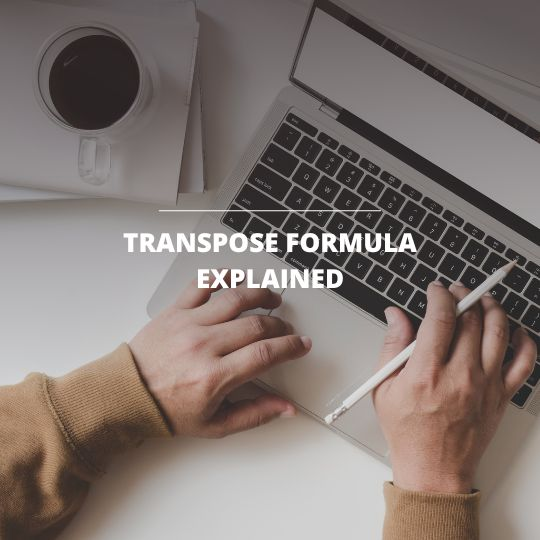# Transpose Formula Explained28
November
2022### How to Use Transpose Formula?

Remember the time when you receive a report from your colleague, which has some information related to your market rate? You realize that the report has completely opposite rows and columns with the file you have. You totally freak out: “Gather around everyone, we have a solution for you: Transpose!” In Microsoft Excel, we have the transpose formula, where you can take the transpose of a cell range. In this situation, you have a table with product IDs on the rows and the sales price on the column. When you transpose the table, you will have products on the columns and the IDs on the rows. Basically, transposing is changing the columns with rows and swapping their places. In this blog, we will show you how to transpose a table in two different ways.

### Transpose – Paste Special

The first way to transpose is to copy and paste special the values on a blank area in the spreadsheet. To do transpose, we need to copy the cell range first. Then, we will open the paste special window and select the transpose option from there. Since you copied and pasted the values, remember to change the values if there is any change in the original cells. Let’s walk through the example below together.

In the example below, we first highlighted the cell range A2: B5 and used the shortcut Ctrl + C to copy the cells. Next, we pressed F5 to open the Go To window and select the cell A7 to where we wanted to paste our table. Then, we opened the paste special window with Ctrl + Alt + V and selected Transpose formula by clicking the underlined letter E for transpose. Finally, by pressing enter, we copied the values, took the transpose of the table and pasted in cell range starting from cell A7.

### Transpose Function

Another way to take a transpose of a cell range is using the =Transpose formula in Excel. However, there is a difference between using paste special and transpose function. With the formula, if you change any value in the original cell range, the transposed version will automatically change as well. Therefore, using the formula version of transpose might save you a lot of time in some cases. Let’s take a close look at how to use =Transpose formula with an example.

In the pictures below, you can see that we have the same table in the cell range A2: B5 in our spreadsheet. Before we start typing =Transpose function in cell A7, we need to highlight the range that we want to transpose. For example, since we are going to copy the 4-row x 2-column sized table, we will take the transpose of it. Then, we need to highlight a 2-row x 4-column area starting from A7. The reason is that =Transpose is an array function in Excel. Therefore, we need to highlight the area before we use our function.

After we highlighted the area, we can start typing our formula =Transpose. Since we want the cells in range A2: B5 to be transposed, we type =Transpose(A2: B5) in cell A7. After typing the formula, we will use the shortcut for pasting an array function – Shift + Ctrl + Enter. Finally, you will see the transposed version of the original table in cell range A7: D8.

Transpose formula is an essential tool for most of the business people who are working with Microsoft Excel. It has a great use in business and can save you a huge amount of time. Depending on the case, you can select either way to have the transpose of a table. By self-studying Transpose function in Excel, you can build up your skills and improve your efficiency in Excel.